Reach Us+44-1202068036
Modularization Analysis of Brain Functional Network Using Fuzzy C-means Algorithm and Correlation in Resting State | OMICS International
Journal of Health & Medical Informatics

Like us on:

All submissions of the EM system will be redirected to Online Manuscript Submission System. Authors are requested to submit articles directly to Online Manuscript Submission System of respective journal.

# Modularization Analysis of Brain Functional Network Using Fuzzy C-means Algorithm and Correlation in Resting State

Zhuqing J1,2, Huan W1 and Ling Z1,2*

1School of Information Science and Engineering, Changzhou University, Changzhou 213164, China

2Changzhou Key Laboratory of Biomedical Information Technology, Changzhou University, Changzhou 213164, China

*Corresponding Author:
Ling Z
School of Information Science and Engineering
Changzhou Key Laboratory of Biomedical Information Technology
Changzhou 213164, China
Tel: 0519-86330339
E-mail: [email protected]

Received date: May 11, 2017; Accepted date: May 16, 2017; Published date: May 20, 2017

Citation: Zhuqing J, Huan W, Ling Z (2017) Modularization Analysis of Brain Functional Network Using Fuzzy C-means Algorithm and Correlation in Resting State. J Health Med Informat 8:255. doi: 10.4172/2157-7420.1000255

Copyright: © 2017 Zhuqing J, et al. This is an open-access article distributed under the terms of the Creative Commons Attribution License, which permits unrestricted use, distribution, and reproduction in any medium, provided the original author and source are credited.

Visit for more related articles at Journal of Health & Medical Informatics

#### Abstract

Aiming to study the local functional structure of brain function network in resting state, the Fuzzy C-means (FCM) algorithm is adapted to modular the brain functional network. Then the nodes in module are connected into a network using correlation between the time series extracted from Functional Magnetic Resonance Imaging (fMRI) data. Afterwards node degree, clustering coefficient and shortest path length are used to analyse the functional characteristics of networks. Finally, the differences in activation between patients and normal controls’ brain regions are compared through Amplitude of Low Frequency Fluctuation (ALFF). Experimental results demonstrate that, the shortest path length of the patient is smaller than that of the normal human, so the information transmission rate increases. Clustering coefficient is higher than the normal, and the degree of grouping of network is enhanced. The correlation between the patient nodes is generally greater than the normal, and there is a weakened situation in the local area. It also found that the proportion of region for the activation level higher than the average of the whole brain in normal with more than the patient. In particular, the activation level of the Precentral gyrus (PreCG) and other regions in patient has a large degree decline. And the activation level in left Caudate nucleus (CAU.L), the lenticular nucleus, Putamen (PUT) and the lenticular nucleus, Palladium (PAL) and other regions is increased for patient. The research results verify the feasibility of modularization analysis of brain functional network using algorithm and correlation in resting state.

#### Keywords

Brain functional network; Fuzzy C-means (FCM); Module; Correlation

#### Introduction

The human brain is one of the most complex systems of nature, and neuronal cells are connected together by Synapses to form a complex brain structure network. The spontaneous activity and the excitement or inhibition of external stimuli in neuronal cells are also transmitted through synapses to other neurons, so that the brain can be coordinated with each other when the body undergoes physiological activities, such as languages, perception and emotion. In order to study the functional structure of the brain more clearly in resting state, researchers constructed brain functional network using Electroencephalography (EEG), Magneto Encephalography (MEG), Functional Magnetic Resonance Imaging (fMRI) and other technology. And the working mechanism in brain is researched combined with complex network analysis methods. Until now, many studies shown that the brain network has the characteristics of modular structure [1-3] found that the human cortical thickness network had an organizational pattern corresponding to brain functional modules (such as vision, language, etc.). It also found that the network can be divided into sub-function modules such as visual network, auditory network and default network . With the deepening of the study, the modular structure has become a focus on researching the local functional structure of brain network.

Nowadays, there are many algorithms for dividing brain networks into modules, such as Kernighan-Lin, GN, clustering and others [5,6]. For example, the components of brain were partitioned using a greedy algorithm and then it found that the visual module existed during the resting state . On the basis of the Newman algorithm, researchers performed a module analysis of the brain functional network with young and old people in resting state . Among them, clustering is an unsupervised machine learning method to partition a collection of multivariate data points into meaningful clusters, where all members within a cluster represent similar characteristics and two data points between different clusters are dissimilar to each other . The similarity criterion for distinguishing the difference between data points is generally measured by distance [10-12]. Data points belong to the same group if they are much closer to each other, while they are evidently from different groups if the distance between them is distinctly large [13,14]. The fuzzy clustering determines the degree of each data point belonging to the same group for the degree of membership function. Which is soft partition, and it’s different from other hard clustering methods to classify the data points to certain cluster.

The dynamic activity between different neurons or brain regions can be described intuitively through the brain function network, and the connection between nodes indicates the dynamic coordination between neural signals. In this paper, the fuzzy c-means clustering algorithm is used to partition the brain function network of patients with Parkinson and normal human. After the partition is completed, the module closely related to the patient is selected to study. Afterwards, the undirected network is constructed by correlation in module, and the functional structure of nodes and networks are analysed. Furthermore, the differences in activation of the specific brain region are studied through the statistical results of the Amplitude of Low Frequency Fluctuation (ALFF).

#### Materials and Methods

The brain fMRI data of patients and normal human were collected with Siemens 3.0 MAGNETOM Trio Tim. The scanning parameters of the device are set as follows. Functional Image: Axial Slices=24, Layer Thickness=4 mm, Repeat Time TR=2000 ms, Echo Time TE=35 ms, angle flip=90°, FOV=230 × 182 mm. Structure Image: 3D Sequence Number=270, Layer Thickness=0.6 mm, Repeat Time TR=7.4 ms, Echo Time TE=3.4 ms, Angle Flip=8°, FOV=250 × 250 mm. Before brain functional network are constructed, the experiment data must be preprocessed.

The brain fMRI images are pre-processed by toolbox of SPM8 (Statistical parametric mapping) and REST (Resting-state fMRI data analysis toolkit) in Matlab. It includes slice time, realignment, normalization, smooth and filtering, the filtering rang is 0.01-0.08 Hz. Then, according to the AAL (Anatomical Automatic Labelling) partition template, the brain is partition into 90 brain regions, the left and the right brains are divided into 45 regions respectively, and match with the pre-processed fMRI images. Finally, the time series of brain regions are extracted from fMRI data by DPARSF (Data processing assistant for resting-state fMRI). In order to build the N × N (N=90) connectivity matrix C (Figure 1a), the correlation coefficient of the time series between any two brain regions is calculated. The correlation coefficient r is defined as: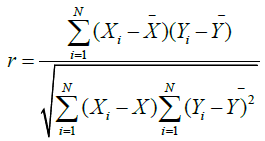(1)

Where Xi and Yi represents the time series of node X and Y, X and Y represents the mean time series of node X and Y, respectively.

The threshold is selected to binarize the correlation matrix C, so we can get a binary adjacency matrix A (Figure 1b). Elements below the threshold are set to zero; surviving elements can either be set to one, as follows: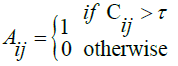(2)

Where τ represents the threshold. According to the matrix C, the brain functional network is partitioned into some reasonable modules using fuzzy c-means, and the undirected networks are constructed in module (Figure 1a and 1b).

The main idea of the k-means is the minimization of an objective function, which is normally chosen to be the total distance between all patterns from their respective cluster centres. Its solution relies on an iterative scheme, which starts with arbitrarily chosen initial cluster memberships or centres. The distribution of objects among clusters and the updating of cluster centres are the two main steps of the k-means algorithm . The algorithm alternates between these two steps until the value of the objective function cannot be reduced anymore.

FCM Clustering is a soft version of k-means, where each data point has a fuzzy degree of belonging to each cluster . The FCM algorithm for vector set is a clustering technique that aims to partitioning a set of measured vector xi (i=1,2,...,n) into Gi (i=1,2,...,c) clusters, the main result is the minimization of an objective function J(U,G) with respect to a fuzzy partition matrix U and a set of prototypes G through cluster centre of each cluster.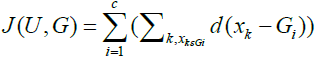(3)

Where d (xk-Gi) represents a universal distance function. Corresponding to the fuzzy partition, elements value of U=[uij]c×n is allowed 0 to 1. However, with the normalization rule, the membership of cluster is equal to one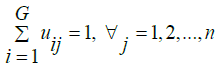(4)

Where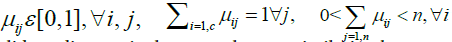When the Euclidean distance is chosen as the non-similarity the vector xk of cluster i and the corresponding cluster centre Gi, the objective function can be defined as: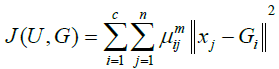(5)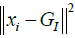denotes the Euclidean distance between the jth vector and the ith cluster centre, also other distance measures could also be used. Where m (1 < m<∞) is the controller of fuzziness (e.g., m=1) means hard partition and m=∞ means completely fuzzy, the value of m is 2 without special requirements. Constructing a new objective function, it can find the necessary condition of the Eq. (5) reach to minimization.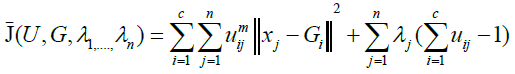(6)

Where λj (j=1,2,...,n) is Lagrange multiplier of the n constrained expressions of Eq. (4), and it takes the derivative of all input quantities in Eq. (6), the requirement for minimization of Eq. (5) is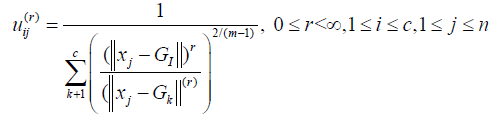(7)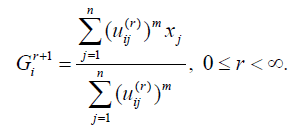(8)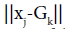in this indicates the Euclidean distance between the jth vector and kth cluster center, the initial value of r is zero and Gk is the centre of cluster k.

According to the above definition, FCM algorithm can be briefly described as follows:

(1) Randomly select a set of c initial centres G.

(2) Compute the partition matrix U using Eq. (7).

(3) Update the centres of all clusters using Eq. (8).

(4) Compute the new objective function J using Eq. (6).

(5) Repeat steps (2) to (4) until New Old New Old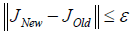.

Centrality is used to determine the role of each node, and the node with the largest centrality is hub in the module. Degree centrality measure the centre degree of node using node degree, and betweenness centrality measure the centre degree of node in module by information flow. If the module network has n nodes, the node degree of vi is wi, the out-degree and in-degree of vi is viout and wiin , respectively.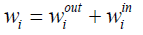(9)

The betweenness centrality of the node vi is defined as: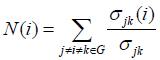(10)

Where σjk represents the quantity of shortest path length from node vj to vk, σjk (i) represent the quantity of shortest path length of passing through node vi. The greater of centrality, which indicates the function connection strength, is relatively strong, and the node is hub in the module.

The shortest path length can be used to analyse the transmission efficiency of information in network. It describes the internal structure of the network and plays an important role for information transmission. The shortest path length lij from node vi to node vj is defined as the minimum number of edges to experience from vi to vj, the reciprocal 1/lij is the efficiency from node vi to vj, denoted as Cij, then the efficiency of the module Gc is defined as: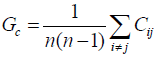(11)

The shorter of shortest path length, so the faster of information transfer rate, and the efficiency of the network is relatively higher. Through the clustering coefficient of network, it is helpful to study the local characteristics of module. In general, a network with high clustering coefficients and shortest path length shows small world effect. In module G, if the node vi is connected to ki nodes, the maximum number of edges between ki nodes is ki (ki-1)/2, denoted as ni. Then the clustering coefficient Ci of the node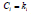ins defined as: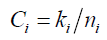(12)

Where ki represents the node degree of vi, the average clustering coefficient C of the module is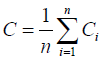(13)

#### Results

The matrix C is regarded as N=90 rows vector xi (i=1,2,..,90) or column vector, these vectors are classified into c groups with nonsimilarity index with Euclidean distance. In general, the value of c is much smaller than the number of samples, but it is greater than one. Because of the randomness of FCM, after several partitions, the brain function network is divided into relatively stable eight modules, as shown in Table 1.

Module Node Quantities Regions (L: Left; R: Right)
1 6 Superior frontal gyrus, orbital part (R), Gyrus rectus (L), Posterior cingulate gyrus (L), Superior occipital gyrus (L), Inferior occipital gyrus (R), Fusiform gyrus (L)
2 9 Superior frontal gyrus, orbital part (L), Middle frontal gyrus, orbital part (R), Insula (L), Amygdala (L), Heschl gyrus (R), Superior temporal gyrus (L), Middle temporal gyrus (R), Temporal pole: Middle temporal gyrus (R)
3 10 Precental gyrus (L, R), Superior frontal gyrus, dorsolateral (L, R), Middle frontal gyrus (L, R), Supplementary motor area (R), Superior frontal gyrus, medial (L, R), Postcentral gyrus (R)
4 10 Inferior frontal gyrus, opercular part (R), Inferior frontal gyrus, triangular part (L, R), Supplementary motor area (L), Anterior cingulate and paracingulate gyri (R), Median cingulate and paracingulate gyri (L, R), Postcentral gyrus (L), Paracentral lobule (L, R)
5 12 Inferior frontal gyrus, opercular part (L), Anterior cingulate and paracingulate gyri (L), Superior parietal gyrus (L, R), Inferior parietal, but supramarginal and angular gyri (L, R), Angular gyrus (L, R), Precuneus (L, R), Inferior temporal gyrus (L, R)
6 13 Middle frontal gyrus, orbital part (L), Rolandic operculum (L, R), Superior frontal gyrus,medial orbital (L, R), Parahippocampal gyrus (L, R), Supramarginal gyrus (L, R), Caudate nucleus (L, R), Heschl gyrus (L), Temporal pole: Middle temporal gyrus (L)
7 15 Inferior frontal gyrus, orbital part (L, R), Insula (R), Hippocampus (L, R), Amygdala (R), Lenticular nucleus, putamen (L, R), Lenticular nucleus, pallidum (L, R), Thalamus (L, R), Superior temporal gyrus (R), Temporal pole: Superior temporal gyrus (R), Middle temporal gyrus (L)
8 15 Olfactory cortex (L, R), Gyrus rectus (R), Posterior cingulate gyrus (R), Calcarine fissure and surrounding cortex (L, R), Cuneus (L, R), Lingual gyrus (L, R), Superior occipita gyrus (R), Middle occipital gyrus (L, R), Inferior occipital gyrus (L), Fusiform gyrus (R)

Table 1: Node distribution in the modules of patients.

The brain function network of the normal group was divided into the same eight modules as the patient, and the brain region in each module is the same as the patient. It was found that the brain region of strong correlation with Parkinson’s disease, such as Precental gyrus (PreCG), Caudate nucleus (CAU), Lenticular nucleus, putamen (PUT) and other regions are mostly distributed in modules 3, 5, 6 and module 7 [17,18]. Then, the Pearson correlation coefficient between any two brain regions is calculated. Due to the global threshold removes some of the connections, so the local threshold is chosen to binarize the correlation coefficient matrix. The threshold is selected from 0, and the step size is 0.05, and the network must be a connected network with no isolated nodes. Taking the module as a whole, the shortest path length of each module is analysed; we can understand the information transfer speed in the module, as shown in Figure 2.

As seen from Figure 2, when the local thresholds in modules 3, 5, 6, and 7 are greater than 0.3, 0.15, 0.16 and 0.25 respectively, the shortest path length does not exist in the four normal modules, and the network is a non-connected network. To ensure that the network is connected, the critical value is selected to binarize the local correlation coefficient matrix, and the thresholds are 0.3, 0.15, 0.16, and 0.25 respectively. The network structure is shown in Figures 3 and 4.

It is found that the shortest path length of patient’s network is smaller than that of the normal human, which indicate that the transmission rate of the information in these modules is higher than that of the normal. When the shortest path length in each module of patient and normal person is analysed separately, the differences of information transmission rate can be understood from a smaller range. The shortest path length of normal module 3 is 2.5333, and in module 6, 5 and module 7, they are 2.1795, 1.8485 and 1.8095 respectively. Which indicates that the transmission rate of the information in module 3 is the slowest, and the transfer speed in other modules have different degree of increases. In module 7 of patient, the shortest path length is 1 and it is the smallest, so that there is the largest information transmission speed in the module. The shortest path length in module 3, 5 and 7 are 1.3778, 1.5758 and 1.5897 respectively, it indicates that the transmission rate is relatively smaller for these modules.

When the threshold is greater than the critical value, the shortest path length of patient still exist, it shows that the correlation in the same module between patient’s nodes is generally lower than normal. The study also found that the critical values in modules 5 and 6 were smaller than those of modules 3 and 7, which indicates the overall correlation of modules 5 and 6 are less than that of the other two modules.

The clustering coefficient of the brain function network refers to the average of the clustering coefficients of all the nodes in the network. It can be seen as the grouping degree of the module, as shown in Figure 5.

As shown in Figure 5, the analysis of the network from patient and normal, it found that the clustering coefficients of normal are 0.5333, 0.5417, 0.5769 and 0.6489 respectively, and they are 1, 0.6195, 0.7366 and 1 of patient respectively, we can see that the degree of grouping of patient is higher than normal, it indicates that there is a higher connection density of patient network. Through the clustering coefficient in module 3 and 5, it can be found that the grouping level of patient is always greater than that of normal. When the network is not connected, the clustering coefficient in patient module 6 is less than normal in a certain threshold range, which indicates that the clustering coefficient of some nodes in this module of normal human is increased. It is also found that the down trend of clustering coefficient is in the same of normal and patient’s module 7, this shows that there is no significant difference between patient and normal group. Therefore, the grouping degree of module 3 and module 7 is the largest and the same, and the other modules have a relatively small degree of grouping. When these modules are connected, the clustering coefficient of the patients is higher than that of the normal, and the shortest path length is smaller than normal human, indicating that the small world effect is more obvious.

According to the degree centrality, it is helpful to determine the functional connection strength and status of nodes in network. The greater of degree centrality, it means that the degree of effect of the node in the network is stronger, which is the hub for the network, as shown in Figure 6.

Through Figure 6, the node degree is the same in module 3 of patient. It shows that the function connection strength of these nodes is consistent. The node SFGdor.L and SFGdor.R have the largest node degree in module 3 of normal group, and they are the same, which indicates their functional connection strength is relativity stronger, and they are the hub in the network. In patients’ module 5, the node IPL.L has the largest node degree, so the degree of effect is the largest, which is the hub. In the normal group module 5, node IPL.R, ANG.L, and ANG.R have the same node degree, it represents that the degree of effect of these nodes is in the same. In module 6, the node degrees of node SMG.L and CAU.L are the largest of normal and patient, respectively, which shows that they are the hub and have the larger functional connection strength. The node degree of INS.R, HIP.R, AMYG.R, PUT.L and PAL.R in normal module 7 are the same and have the maximum, so the degree of function connection of these nodes is the same, and the node degree of each node in patient module 7 is equal.

It is also found that there is a large change of node degree of some regions, which include PoCG.R in module 3, IPL.L and PCUN.R in module 5, CAU.L in module 6, ORBinf.R and MTG.L in module 7. The node degree of these nodes is increased, which indicates that the functional connection strength is enhanced, and there is an important role during the transition from normal to patient. In addition, the node degree in the patient module 3 and module 7 is greater than normal group, it represents that the functional connection strength in the two modules is greater than normal.

#### Discussion

Usually, the activation level of brain regions is studied using ALFF method, the size of the ALFF value corresponds to the strength of the Blood Oxygen Level Dependent (BOLD) signal. When the ALFF of one brain regions is increased, it indicates that the activity of neurons increases, the energy distribution is larger. On the contrary, if the low-frequency amplitude decreases, the neurons’ activity is small, the energy distribution also decreases [19,20]. One sample t test is performed on the ALFF values of the patient group and the control group, so the deviation statistics (t value) of activation level between the brain of a region and the average value of the whole brain can be obtained. It shows the largest statistical difference between a region of the brain and the whole brain, as shown in Figure 7.

Figure 7: Statistical properties of ALFF and energy distribution in special region for patients and normal human. (a) The statistics result of ALFF for normal human; (b) The statistics result of ALFF for patiens; (c) Energy distribution and t value of PCG.R in normal human; and (d) Energy distribution and t value of PCG.R in patient.

In Figure 7, the red areas represents that the activation level of the brain region is higher than the average value of the whole brain in resting state. By the distribution of the red regions, it can be seen that the activation region for normal person is significantly more than patient, which indicates that in the course of the transition from normal human to patient, the activity of some regions changed a lot. From the statistical results, we found that the activation level of some regions is decreased significantly [21-25], such as PCG.R, which indicates the functional connection in the region is abnormal, and the range of energy distribution is also quite different. Similarly, the two sample paired t test (P<0.05) is performed on the ALFF value of the patient group and the normal control group. The nodes with large change of node degree and the brain regions with strong correlation of patients were selected as a module, the statistical result as shown in Table 2, the network structure of different threshold as shown in Figure 8.

Brain region Abbreviation Left/Right Montreal Neurological Institute (MNI) t value
X Y Z
Inferior frontal gyrus, orbital part ORBinf Left -36 30 -12 -0.57
Postcentral gyrus PoCG Right 42 24 54 2.79
Inferior parietal, but supramarginal and angular gyri IPL Left 42 45 48 4.75
Precuneus PCUN Right 9 -57 45 7.81
Caudate nucleus CAU Left -12 12 9 -0.59
Middle temporal gyrus MTG Left -57 -33 -3 -0.33
Lenticular nucleus, putamen PUT Left -24 3 3 -0.58
Lenticular nucleus, putamen PUT Right 27 6 3 -0.57
Lenticular nucleus, pallidum PAL Left -18 0 0 -0.65
Lenticular nucleus, pallidum PAL Right 21 0 0 -0.47
Precental gyrus PreCG Left -39 -6 51 7.24
Precental gyrus PreCG Right 42 -9 51 3.83
Posterior cingulate gyrus PCG Left -6 -42 24 2.51
Posterior cingulate gyrus PCG.R Right 6 -42 21 -0.02

Table 2: Statistical properties of ALFF of brain regions.

Figure 8: Network structure and node degree of patient and normal human. (a) Network structure of patient; (b) Network structure of normal; and (c) Node degree distribution of patients and normal controls.

If the statistical value is greater than 0, it indicates the activation level of patient is higher than that of the normal. Otherwise, the activation level is lower than that of the normal. As seen from Table 2, the t value of node ORBinf.L, CAU.L, MTG.L, PUT, PAL and PCG.R are less than 0 in patients, which indicates that activation level of these regions are less than those in normal group, and the functional connection strength of patient is relatively small. The value of node PoCG.R, IPL.L, PCUN.R, PreCG and PCG.L are greater than 0, this shows that the activation of this part of the patient’s [26-30] region is higher than the normal, and the functional connection strength is relatively large. As can be seen from Table 2, the Precuneus’s statistical value is large, which shows that the degree of activation in the region has a greater degree of weakening of patient.

It can be seen from Figure 8, the node degree of ORBinf.L, PCG.L, PCG.R, PoCG.R, PCUN.R, PUT.L, PAL.L and node MTG.L in patients is greater than those of the normal person, which indicates the functional connection strength of these nodes is greater than controls. Furthermore, from the statistical results of the ALFF value, it can be seen that the statistical value of node ORBinf.L, PUT.L, PCG.R, PAL.L and MTG.L are less than 0, so we think that the degree of effect for these nodes is increased in the local area. Node degree of IPL.L and CAU.L node is less than normal, so the degree of functional effect is less than normal. However, the statistics value of node IPL.L is [31-33] greater than 0, which indicate that the function connection strength of this region is increased in whole brain, but the experimental results show that in the local area of decline. Similarly, the same is true for the four modules selected, such as in patient module 7, node degree of ORBinf.L is greater than normal, we can believe that the correlation is relatively weak between the region and other regions, while normal human is enhanced.

In this paper, the FCM algorithm was used to divide the brain function network into modules by the correlation coefficient. The undirected networks were analysed by correlation and ALFF. The information transfer rate and the degree of grouping of the network are analysed using the shortest path length and the clustering coefficient respectively. The function connection strength and status of each node are studied by the node degree. To understand the distribution activation level of brain regions, the method of ALFF is selected to analyse the difference between patient and normal. Finally, the results of the two groups were compared, due to the severity degree of the disease and randomness with other factors, the need for continuous follow-up analysis, resulting in ubiquitous results.

#### Acknowledgements

The authors would like to thank the reviewers and the editors for their valuable comments and suggestions on improving this paper. This work is supported by the National Natural Science Foundation of China (No. 51307010 and No. 61201096).

#### References

Select your language of interest to view the total content in your interested language

### Article Usage

• Total views: 1279
• [From(publication date):
May-2017 - Jul 18, 2019]
• Breakdown by view type
• HTML page views : 1203Can't read the image? click here to refresh